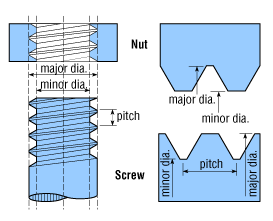# Metric Conversion Guide

## The Metric Conversion Guides Includes:

• Conversion Factors for Length, Area, Volume, and Mass
• Metric Prefixes
• AWG to Metric Wire Sizes
• Temperature

### Conversion Factors for Length, Volume, Area, and Mass

Metric units are used for most linear, weight, and temperature measurements, as well as for thread descriptions:

#### Length

Inch x 25.40 = Millimeters
Millimeters x 0.03937 = Inches
Feet x 0.3048 = Meters
Meters x 3.281 = Feet
Miles x 1.609 = Kilometers
Kilometers x 0.6214 = Miles
Ohms/Kilometer x 0.3048 = Ohms/1000 ft.
Ohms/1000 ft. x 3.2808 = Ohms/Kilometer

#### Volume

Cu. Inch x 16.39 = Cu. Centimeter
Cu. Centimeter x 0.06102 = Cu. In.
Cu. Foot x 0.02832 = Cu. Meter
Cu. Meter x 35.31 = Cu. Foot

#### Area

Sq. Inch x 6.452 = Sq. Centimeter
Sq. Centimeter x 0.1550 = Sq. Inch
Sq. Foot x 0.0929 = Sq. Meter
Sq. Meter x 10.76 = Sq. Foot
Sq. Mile x 2.590 = Sq. Kilometer
Sq. Kilometer x 0.3861 = Sq. Mile
Circular Mil x 1,000,000 = Circular In.
Circular Mil x 0.7854 = Sq. Mil

#### Mass

Ounce x 28.35 = Gram
Gram x 0.03527 = Ounce
Pound x 0.4536 = Kilogram
Kilogram x 2.205 = PoundMost threaded components and components with mounting holes (such as AC power inlets) listed in this catalog are referred to in terms of their metric dimensions. For example, an M3 mounting hole indicates that the hole is sized for an M3 mounting screw. The metric screw will be referred to in the following format:

M3 X 0.50

As these drawings illustrate, M3 refers to a major diameter of 3mm. The pitch or distance between threads is 0.50mm.

AWG to Metric Wire Sizes Conversion Chart
AWG Equivalent in mm2
20 0.519
18 0.824
17 1.04
16 1.31
15 1.65
14 2.08
13 2.63
12 3.31
11 4.17
10 5.26
9 6.63
8 8.37
7 10.6
6 13.3
5 16.8
4 21.2
3 26.7
2 33.6
1 42.4
Metric Prefixes
Tera 1012 T
Giga 109 G
Mega 106 M
Kilo 103 k
Hecto 102 h
Deca 101 da
Deci 10-1 d
Centi 10-2 c
Milli 10-3 m
Micro 10-6M µ
Nano 10-9 n
Pico 10-12 p